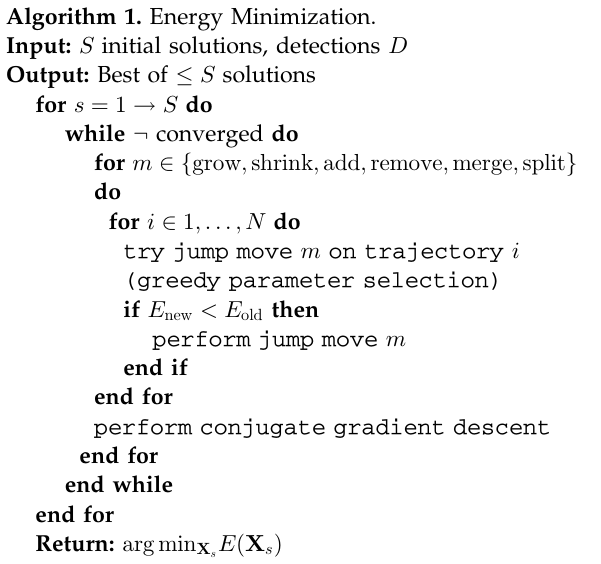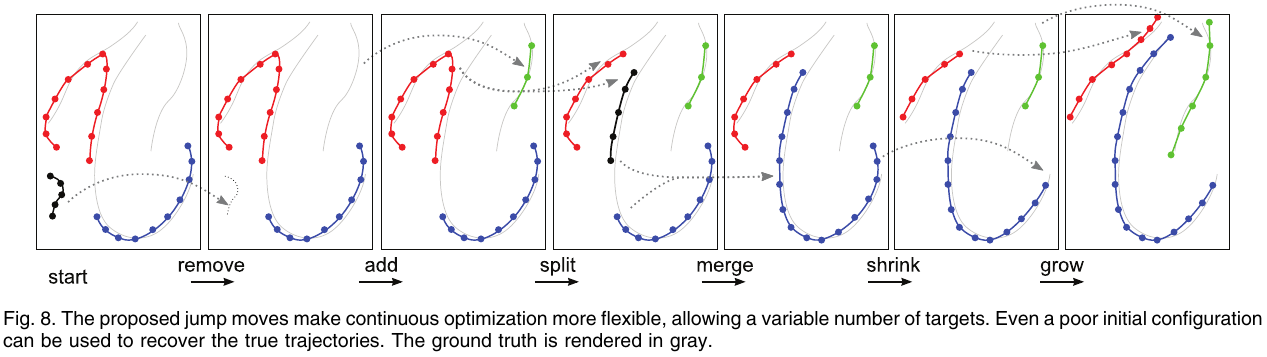# Multiple Object Tracking via Minimizing Energy

##### Posted on Jul 05, 20210 Comments

Several recent multitarget tracking formulations aim to obtain a (nearly) globally optimal set of trajectories within a temporal window. To make (near) global optimization possible and efficient, the state space is reduced by restricting the possible target locations to a finite set and the energy is simplified.

The energy depends on the locations and motions of all targets in all frames, including cases where image evidence is missing, and explicitly includes physical constraints, such as smoothness of motion and mutual exclusion.

• recursive methods:
• Kalman filter approaches
• particle filtering (also known as sequential Monte Carlo)
• nonrecursive methods:
• all trajectories are estimated jointly within a given time window, but the solution space is restricted to a finite number of states

the common objective of energy minimization methods is to - set up a function that assigns every possible solution a cost (the “energy”) and then (approximately) find the state with the lowest cost.

In computer vision, one often faces two major problems: - the input data is noisy and requires robust models - an accurate representation that captures all relevant nuances of the real situation quickly becomes very complex

a dilemma: should the energy function be simplified until it is easily optimizable, or should it rather have the power to capture the complex situation, at the cost of less graceful mathematical properties?

There energy function is a linear combination of six individual terms:

$E = E_{det} + \alpha E_{app} + \beta E_{dyn} + \gamma E_{exc} + \delta E_{per} + \epsilon E_{reg}$

where

The aim is then to find the state $X^\star$ that minimizes the high-dimensional continuous energy form:

$X^\star = \arg\min_{X\in \IR^d}E(X)$

Likely pedestrian locations are found with a sliding-window linear SVM detector. - the features employed in the detector include histograms of oriented gradients (HOG) and histograms of relative optic flow (HOF) - detection peaks are found by nonmaxima suppression (NMS) and projected onto the ground plane of the world coordinate system, where they form the image evidence for tracking.

• observation model: the energy should be minimal when the location of each target precisely matches a detection. To capture the localization uncertainty of the object detector, the energy smoothly increases with the disease between the estimated object location $X_i^t$ and a detection location $D_g^t$.
• dynamic model: objects move slowly relative to the frame rate, and in most cases also smoothly. use a simple constant velocity model that minimizes the distance between consecutive velocity vectors
• mutual exclusion: apply a continuous penalty to configurations in which two targets come too close to each other
• trajectory persistence: encourage trajectories to start and end along image borders or along a predefined perimeter
• regularizer: prevent the number of targets from growing arbitrarily large so as to better fit the data

## Optimization

Use the standard conjugate gradient method. Upon convergence, a jump move is executed (unless it would increase the energy), which may change the dimensionality of the models. The jumps give the optimization a high degree of flexibility–the initial solution need not even have the correct number of targets.• growing and shrinking
• merging and splitting
• adding and removing: the newly inserted tracks are started conservatively with three consecutive frames

Like any nonconvex optimization, the result depends on the initial value from which the iteration is started. They propose to use the output if an arbitrary simpler tracker as a more qualified initial value. In their experiments, they used per-target extended Kalman filters (EKFs) where the data association is performed in a greedy manner using a maximum overlap criterion.

Published in categories Note